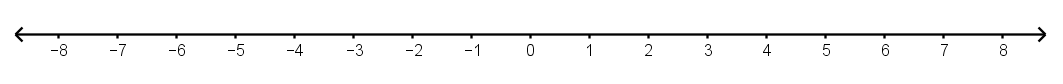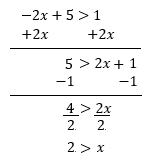# Equations and Inequalities

## Objective

Solve inequalities with negative coefficients.

## Common Core Standards

### Core Standards

?

• 7.EE.B.4.B — Solve word problems leading to inequalities of the form px + q > r or px + q < r, where p, q, and r are specific rational numbers. Graph the solution set of the inequality and interpret it in the context of the problem. For example: As a salesperson, you are paid $50 per week plus$3 per sale. This week you want your pay to be at least \$100. Write an inequality for the number of sales you need to make, and describe the solutions.

?

• 6.EE.B.5

• 6.EE.B.8

## Criteria for Success

?

1. Understand that solving a corresponding equation to an inequality will give the value for the variable where the solutions begin; testing solutions on either side of this value will indicate the direction of the solutions to the inequality.
2. Understand that in solving an inequality with a negative coefficient, the variable term can be added to both sides and solved with a positive coefficient; in this case the relationship of the variable to the inequality symbol has flipped. For example, the solution to $-2x<6$ is not values for $x$ that are less than $-3$, but rather values for $x$ that are greater than $-3$.
3. Solve inequalities with negative coefficients.

## Tips for Teachers

?

• In Lesson 10, students encounter inequalities with negative coefficients and must make sense of an unexpected solution. The three Anchor Problems guide students through different approaches to understanding these problems conceptually rather than as a trick of “flipping the inequality symbol.”
• A common misconception that happens if students learn only the rule and not the conceptual understanding behind it is to over-apply the rule whenever there is a negative in the problem. For example, a student who does not understand this concept may solve ${3x>-9}$ and ${-3x>9}$ the same way as ${x <-3.}$ If the student were to test out possible values for the solution into the inequality, the student would see that this is the solution to only one of those inequalities.

#### Fishtank Plus

• Problem Set
• Student Handout Editor
• Vocabulary Package

## Anchor Problems

?

### Problem 1

In the chart below, one equation and three inequalities are shown. For each one, write the solution and use the space in the last row to check your solution.

 Inequality/Equation $3x = 12$ $3x < 12$ $3x < - 12$ $- 3x < 12$ Solution Check solution

What challenges arise with the inequality ${-3x<12}$? Is this what you expected to happen?

### Problem 2

Ralph is trying to solve the inequality ${-{ 1\over 3}(x-48)\leq15.}$ He knows how to solve equations, so he writes the corresponding equation ${-{ 1\over 3}(x-48)=15.}$

1. Solve Ralph’s equation and plot the point on the number line below. How is the solution to the equation related to the solution to the inequality?
2. Pick a number either to the right or to the left of the point you drew on the number line. Test this number as a solution to the inequality. What does this tell you about the direction of the solutions to the inequality?
3. Write the solution to the inequality using an inequality symbol.### Problem 3

Rakiah is solving the inequality -${2x+5>1.}$ She is unsure how to treat the negative 2 in the inequality, so she uses the addition property of equality to rewrite her inequality. See her work below.## Problem Set

?The following resources include problems and activities aligned to the objective of the lesson that can be used to create your own problem set.

?

Match each inequality to one of the solutions. Justify that your solution is correctly matched to your inequality.

Inequalities:

${-10-6x>26}$           ${-10+6x>-46}$

Solutions:

${x>-6 }$          ${x>6}$          ${ x<-6}$           ${x<6}$

?